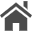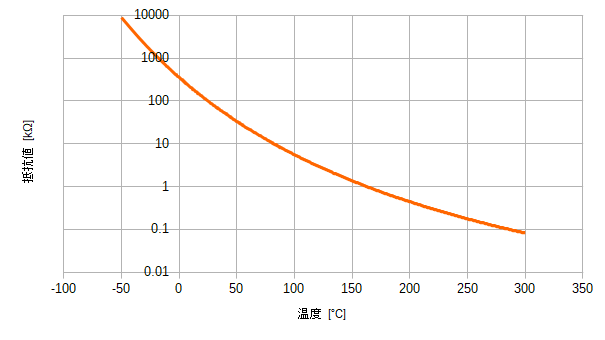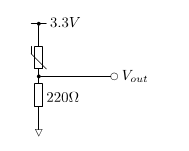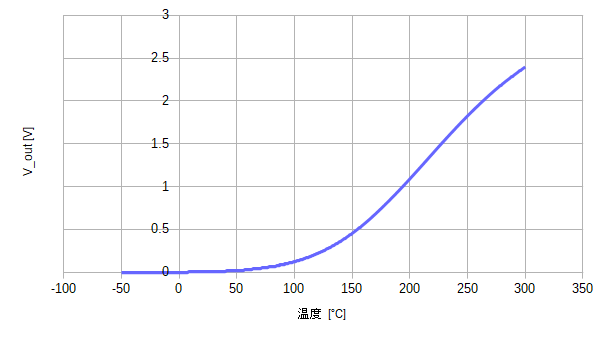Home  Updated: December 05, 2020  [日本語 / English]

# NMeasuring the temperature using the NTC thermistor

## Introduction

An NTC thermistor is an element whose resistance decreases with increasing temperature. With its characteristics, the temperature can be measured.

## The relationship between temperature and resistance

I used a thermistor cartridge from E3D Online. The relationship between temperature and resistance is given in the table. The figure is shown below.## Measurement Circuit

Consider a circuit like the one below to convert the resistance value to a voltage. As the temperature increases, the resistance of the NTC thermistor decreases, so the output voltage $$V_{out}$$ becomes higher.## The relationship between temperature and voltage

The relationship between temperature and $$V_{out}$$ is shown in the figure below. The shape of the curve changes depending on the resistance of the combined resistor, so choose the right resistance value. In this case, we want to measure in the vicinity of 200-250 degrees Celsius, so we chose a resistance value (220 Ω) that would cause a large change in voltage in that range.The output voltage of the measurement circuit is input to the microcontroller's ADC, and the temperature can be obtained from the relationship between temperature and voltage.